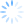`Dim i = {1, 2, 3, 4}`

`    Dim l As New List(Of Integer) From {1, 2, 3, 4}        Dim countryRegex As New Dictionary(Of String, Regex) From {          {"USA", New Regex("^[2-9]\\d{2}-\\d{3}-\\d{4}\$")},          {"UK", New Regex("(^1300\\d{6}\$)|(^1800|1900|1902\\d{6}\$)|(^0[2|3|7|8]{1}[0-9]{8}\$)|(^13\\d{4}\$)|(^04\\d{2,3}\\d{6}\$)")},          {"Netherlands", New Regex("(^\\+[0-9]{2}|^\\+[0-9]{2}\\(0\\)|^\\(\\+[0-9]{2}\\)\\(0\\)|^00[0-9]{2}|^0)([0-9]{9}\$|[0-9\\-\\s]{10}\$)")}          }`